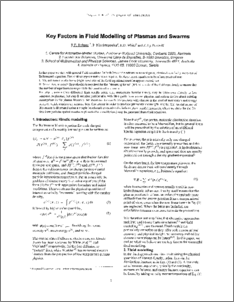# Key Factors in Fluid Modelling of Plasmas and Swarms

Robson, R.E., Nicoletopoulos, P., White, R.D., and Petrovic, Z.Lj (2006) Key Factors in Fluid Modelling of Plasmas and Swarms. In: Proceedings of the 13th Asian Conference on Electrical Discharge. From: ACED2006 13th Asian Conference on Electrical Discharge, 16-19 October 2006, Sapporo, Japan.Preview
PDF
Download (405kB)

## Abstract

In this paper we start with general fluid equations for both ions and electrons in neutral gases, obtained as velocity moments of Boltzmann's cquation. Two distinct approximations are required for these exact equations to be of allY practical use: 1. The collision transfer terms (right hand side of the fluid equations) must be approximated, and II. Some closure ansatz (hypothesis) is required for the "streaming terms" (left hand side of the fluid equations), to ensure that the number of equations corresponds to the number of unknowns. For step I, swarm (frce diffusion) limit results using, e.g., momentum transfer theory, may be taken over directly to low temperature plasmas, but step II remains problematic, with little guide from swarm physics, and serious doubts about existing assumptions in the plasma literature. We focus on electron fluid equations with closure at the level of momentum and energy balance, which requires an accurate heat flux ansatz in order to produce physically meaningful results. The crucial nature of this ansatz is illustrated using a simple benchmark calculation for infinite plane parallel geometry, where we show for the first time how periodic spatial structures (Franck-Hertz oscillations) may be generated from fluid equations.

Item ID: 4605 Conference Item (Research - E1) fluid equation; Boltzmann equation; electron swarms; plasma discharge modelling 17 Jun 2009 02:12 97 EXPANDING KNOWLEDGE > 970102 Expanding Knowledge in the Physical Sciences @ 100% Total: 117 Last 12 Months: 1 More Statistics

Actions (Repository Staff Only)Item Control Page